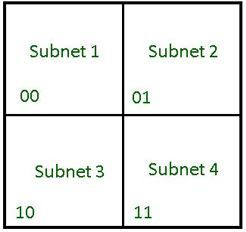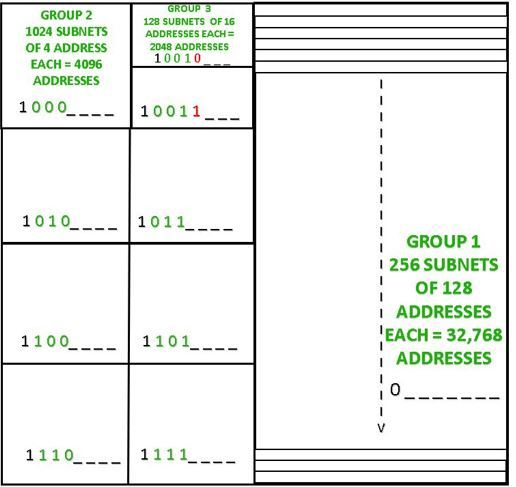# Fixed Length and Variable Length Subnet Mask Numericals

Before starting off with this article make sure you know the basics of Subnetting and Classless Addressing.

When a block of addresses is divided into subnets all having an equal number of addresses, the type of subnetting is said to be Fixed Length Subnetting. The subnet masks used here will be the same for all the subnets as the number of addresses is equal for each subnet.

Example –
Consider an address block 121.37.10.64 /26.
Find the first and last addresses for each subnet, if the number of equal sized subnets required is as given in the input.

Input :

`Number of subnets required = 4 `

Output :

```Subnet-1:

Subnet-2:

Subnet-3:

Subnet-4:

Since 4 subnets are required, we need 2 bits to identify each subnet. Thus the subnet mask now becomes (/28)..
Number of variable bits left = 32 -28 = 4 bits. Thus the total number of addresses in each subnet= 24= 16.Figure – Address space divided into 4 equal sized subnets

There are two ways of approaching the solution:
Method-1:

1. To find the First Address, understand that the first 26 bits will be the same as the address given because they were fixed. As discussed above the next 2 bits will also be fixed.
2. Out of the 16 addresses of that a subnet holds, to point its first address it is required that all the 4 (non-fixed) bits are 0.
3. So the first address of the 1st subnet =
`121.37.10. 01  00  0  0  0  0 /28 = 121.37.10.64/28.`

For the 2nd subnet =

`121.37.10. 01  01  0  0  0  0 /28 = 121.37.10.80/28`

and so on.

4. To find the last address, add (the number of addresses in the subnet -1) to the first address of that subnet. In this case, add (16-1) to the first address of each subnet. So the last address of the 1st subnet is
` 121.37.10.79/28.`

Method -2 (shortcut):

1. To find the first address of the 1st subnet, carryout AND operation between the address given and the subnet mask of the 1st subnet.
Performing AND operation :

```    01111001   00100101   00001010   01000000/26
AND 11111111   11111111   11111111   11110000/28
-------------------------------------------------------------------------
01111001   00100101   00001010   01000000 /28 ```

Thus, first Address of the 1st subnet

`= 01111001  00100101  00001010  01000000  /28 = 121.37.10.64 /28 `
2. To find the last address of a subnet, perform OR operation between the first address of the subnet and the complement of the subnet mask.

`= 01111001 00100101 00001010 01000000 /28  `

`= 00000000 00000000 0000000 00001111 / 28 `

Performing OR operation we get the last address of the 1st subnet

`= 01111001  00100101  00001010  00001111  / 28 = 121.37.10.79 /28 `
3. Add 1 to the last address of the previous subnet to find the first address of the next subnet.

Note –
When the number of subnets required cannot be computed in the powers of 2, then divide address space in a way that the number of subnets is closest to a number that can solely be represented as a power of 2.

For example, if we need 14 subnets, then since 14 cannot be represented solely in the powers of 2, so we divide address space into 16 equal subnets. Now the respective addresses can be found using the above-mentioned method.

2. Variable Length Subnet Masks :
When a block of addresses is divided into subnets containing different numbers of addresses, type of subnetting is said to be Variable-Length Subnetting.
The subnet masks used here might not be the same for different subnets.

Example:
Consider a block of address with starting address 112.78.0.0/16. Find the first and last addresses for each group, if the number and size of the subnets required by them are as given in the input.

Input:

```Group-1:
256 subnets, each needs 128 addresses

Group-2:
1024 subnets, each requiring 4 addresses

Group-1:
128 subnets, each consisting of 16 addresses ```

Output:

```Group-1:

Group-2:
Last Address : 112.78.143.255 / 20

Group-3:
Last Address : 112.78.151.255 /21 ```

To understand such problems, it is important to understand the procedure to fix the bits in the subnet ID and find the subnet mask for each group of subnets.

`= 232-16 = 65,536 `

Understanding the division of address space,

```Group-1:
Number of addresses = 256 x 128 =  32, 768  ```

Thus, group 1 occupies 1/2 of the addresses in the block. So, 1 bit is fixed to identify this half and the Subnet Mask becomes /17.

Similarly for Group-2, a total of 4 bits are fixed and the Subnet Mask becomes /20.

And, for Group-3, a total of 5 bits are fixed and subnet mask becomes /21.

Then using Method 2 mentioned above, the respective starting and ending addresses can be found.For the sake of understanding the problem, let the block of addresses be represented by a big square which can be further divided into the required subnets. Since the total number of addresses in the block = 65, 536, it can be deduced that the square is made up of 256 x 256 addresses. Observe the division of the address space and fixing of the initial bits in the subnet ID of the address space carefully. The blanks along with 8 more blanks together will be the host ID.

Once the address space is appropriately divided and respective subnet masks found, use the methods above to find the required addresses.

Attention reader! Don’t stop learning now. Get hold of all the important CS Theory concepts for SDE interviews with the CS Theory Course at a student-friendly price and become industry ready.

My Personal Notes arrow_drop_upCheck out this Author's contributed articles.

If you like GeeksforGeeks and would like to contribute, you can also write an article using contribute.geeksforgeeks.org or mail your article to contribute@geeksforgeeks.org. See your article appearing on the GeeksforGeeks main page and help other Geeks.

Please Improve this article if you find anything incorrect by clicking on the "Improve Article" button below.